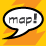# How to recalculate distance from planar to geodesic value

5982
7
Jump to solution
02-05-2015 07:05 AMOccasional Contributor III

Dear Gurus!

It is necessary to find a point at a predetermined distance  in geodesic distance (wgs 84) . How I can do it from planar value?

1 Solution

Accepted SolutionsbyEsri Frequent Contributor

The distorsion between web mercator coordinates and real world distance is: 1/cos(latitude) i.e no distorsion at equator, infinite distorsion at poles.

If you polyline is small enough to consider that the distorsion is constant along the line and that a straight line is an acceptable approximation for a geodesic you could use 1/cos(latitude of the center of your polyline) as ratio.

As likely in your case, it's easier to get the center Y web mercator coordinates than the latitude, you can also define the ratio as:

ratio = Math.Cosh(Y/earthRadius);  with earthRadius approximatively equals to 6378137

7 RepliesbyEsri Notable Contributor

One way is to create a custom projected coordinate system using the azimuthal equidistant projection. The center coordinates are the first point. Calculate the second point using the planar distance (and azimuth). Unproject it.

(sorry, not familiar with Silverlight so I can't be more specific)New Contributor II

Anatolii,

It is hard to know how to answer your question without knowing what you mean by planar distance.  For me, planar distance means a distance that you measured from a map.  It is almost impossible to know what the geodesic distance for such a measurement is without going back and re-measuring it using a geodetically correct method.  If you need to do this, the options are to calculate the distance using the latitude/longitude coordinates of the two points you measured the distance between, to use the geodetic method of the ArcMap measure tool to measure the distance on the map, or to create a custom azimuthal equidistant projection centered on your point of origin and then do the measurement with planar tools.

If your planar distance was specified by a measurement on the ground, then you may need to consult with a geodetic surveyor on the adjustments required to convert the measured distance to a geodetic distance.  I believe the adjustment will be very minor.

If your planar distance was specified externally and not found by measurement, such as "I need to find a location 150,000 meters northeast of the site", then your planar distance probably really is a geodetic distance.

Once you know the geodetic distance you want to find a point for, you may use that distance, with the azimuthal equidistant projection centered on your origin, as a planar distance to create the circle of points that are the correct distance from your point of origin.Occasional Contributor III

I formulated a question badly, sorry. I'll try to reformulate.

There is a road (as polyline layer with WGS_1984_Web_Mercator coordinate system) on the map. And on the 9500 meters from the beginning of the road is some object, which place on the road I must to find (point geometry).  Assume for simplicity - the road is straight.

If I measure the distance between the  kilometer posts, using the tool "Measure" with the "Planar" option, I get the 2314 meters, and with the "Geodesic" option - 1000 meters.  By default in arcmap is used "Planar" option as I use WGS_1984_Web_Mercator , and I manually switch mesure instrument to "Geodesic" option.

Now I try programmatically to define coordinates of the object on the road with using the following expression to calculate the lengths of the segments:

`            public double Length()            {                return Math.Sqrt(Math.Pow(Point2.X - Point1.X, 2) + Math.Pow(Point2.Y - Point1.Y, 2));            }`

I guess that to correctly use the distance of the object from the beginning I must use a conversion ratio (approximatly ration = 2,314 for my case) , ie,

distance = 9500 * ratio ;

If so, how do you know this ratio for a given point of the map?byEsri Frequent Contributor

The distorsion between web mercator coordinates and real world distance is: 1/cos(latitude) i.e no distorsion at equator, infinite distorsion at poles.

If you polyline is small enough to consider that the distorsion is constant along the line and that a straight line is an acceptable approximation for a geodesic you could use 1/cos(latitude of the center of your polyline) as ratio.

As likely in your case, it's easier to get the center Y web mercator coordinates than the latitude, you can also define the ratio as:

ratio = Math.Cosh(Y/earthRadius);  with earthRadius approximatively equals to 6378137Occasional Contributor III

Thanks a lot, Dominique!!!

This is a fragment of your great code (class LinearReferencingExtension). I really enjoyed watching and using it!byEsri Frequent Contributor

Thanks Anatolii,

Glad to know you enjoyed the sample 🙂Occasional Contributor III

P.S. Experimental fact and the correction to the algorithm.

My polyline is actually in Pulkovo 1942 zone 6 , which corresponds to latitude - from 60 to 66 degrees. When I try to calculate road length (with real length 79 045 meters in Pulkovo)   with average values of ratio , I receive 78750 meters. So it is nearly 300 meters distortion.  So now I using next algorithm.

I project road in Pulkovo CS and then find the point on the needed distance. Then I reproject this point to desired CS.